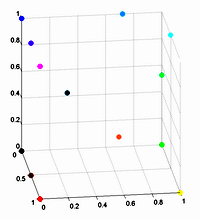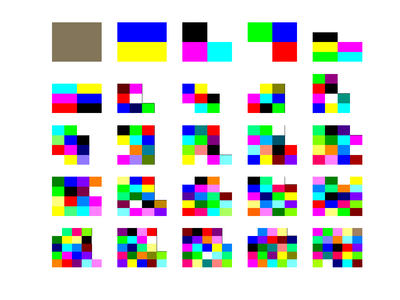## June 19, 2007

### RepulsicolorI recently needed to color a number of objects in such a way to make them as identifiable from each other. The normal colormaps found in Matlab tend to be graded, making it hard to distinguish objects. So I whipped up a little Matlab function to distribute colors "optimally" for maximal distance (included below the fold).

The method treats each color as repulsing the other colors with an inverse hypercubic force. The walls of the RGB cube are impenetrable and force the colors to remain inside.Why not an inverse quadratic force? The reason is that I prefer to get colors in the inside of the cube and not just on the surface: the more slowly decaying low-order forces tend to drive every color onto the surface.

The method works surprisingly well for a reasonable number of colors. Beyond 50 the stepsize is too large to get stabilization, but by now the number of colors is so large that they are not easily identifiable anymore and one could just make a random colormap.

I have tried it in HSV space too by confining points to the HSV color cone. While the method works roughly right, I'm not too pleased with the results. Colors tend to distribute nicely in terms of layers of value and across the top full value circle, but they have no reason to align with the primary colors and this tends to give a muddled look to the colormaps. Maybe it would be worthwhile to try it in the CIE colorspace.

function col=makeoptcolor(N)
% colormap = makeoptcolor(N)
%
%Make colormap with maximal distance between %N colors. Distributes the colors in the RGB cube %using 1/r^4 repulsion. The colormap is %unordered. The method is stable up to about
% 50 colors; beyond that it is simpler to just %generate them randomly.
%
% Anders Sandberg 2007

rand('seed',1);
% Same map is generated each time

r=rand(N,1);
g=rand(N,1);
b=rand(N,1);
h=0.1;

for t=1:100
for i=1:N
dg=g-g(i);
db=b-b(i);
dr=r-r(i);
d=(dg.*dg+dr.*dr+db.*db);

if (sum(d==0)==1)
fr=-sum(dr./(.000001+d).^2)/N;
fg=-sum(dg./(.000001+d).^2)/N;
fb=-sum(db./(.000001+d).^2)/N;

r(i)=r(i)+h*fr;
g(i)=g(i)+h*fg;
b(i)=b(i)+h*fb;

if (r(i)<0) r(i)=0; end;
if (g(i)<0) g(i)=0; end;
if (b(i)<0) b(i)=0; end;
if (r(i)>1) r(i)=1; end;
if (g(i)>1) g(i)=1; end;
if (b(i)>1) b(i)=1; end;
else
r(i)=rand;
b(i)=rand;
g(i)=rand;
end
end
end

col=[r g b];

Posted by Anders3 at June 19, 2007 11:04 AM
Comments